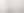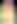## What Is The Difference Between Infinity And Not Defined?Francesca Foster

Go to Case 1

Lets consider the following scenario:

If we had had 8 apples say, we could separate them into four groups of two.  This can be written as

$$\frac{8}{2}=4$$

If we had 8 apples, we could also separate them into two groups of four. This can be written as

$$\frac{8}{4}=2$$

If we had 8 apples, we could also separate them into eight groups of one. This can be written as

$$\frac{8}{1}=8$$

Into how many groups of zero could we separate 8 apples though?

$$\frac{8}{0}=?$$

It doesn't matter how many groups of zero we have, because they would never add up to eight since $$0+0+0+0 +0+0+0+0= 0$$

You could even have one million groups of zero apples and they would still add up to zero. So, it doesn't make sense to divide by zero since there is not a good answer. It is undefined.

Now, consider the number 8.  Divide it by 10 and we get 0.8.  Furthermore,

$$\frac{8}{0.1}=80$$

$$\frac{8}{0.01}=800$$

$$\frac{8}{0.001}=8000$$

etc

So, as the denominator APPROACHES zero, the value of the division becomes larger and larger i.e. when the denominator is so small that is it virtually zero then the division will give you a very large number. We call this very large number infinity. Infinity is a concept, not an actual number. Infinity in itself is well defined -  a number greater than any countable number i.e a value which is greater than any finite value we can specify.

Consider the following 2 undefined cases:

Case 1: Dividing by zero (mentioned at the beginning). Let’s explain this formally now:

take $$\frac{a}{0}$$ which is undefined since a is a non-zero number, real or complex. Let $$\frac{a}{0} = y. \implies a = 0 \times y$$.

No value of y can satisfy this equation because any number multiplied by zero is always zero. So it's undefined because of a lack of solution.

Case 2:  consider $$\frac{0}{0}$$. Is it 0 or $$\infty$$ or 1? It is undefined. Let $$\frac{0}{0} = x. \implies 0 \times x = 0$$.

Any value of $$x$$ (real or complex, will satisfy this equation). So $$x$$ is undefined because of lack of uniqueness. Recall that the algebraic operations of addition and multiplication are binary functions. The result value is always unique. Expressions like $$\frac{0}{0}, \frac{0}{\infty}, \frac{\infty}{0}, 0^{0}, 0^{\infty etc$$ are undefined and known are the indeterminate forms.

Consider $$f(x)=\frac{1}{x}$$. The function is undefined at $$x=0$$. As $$x$$ approaches 0 from the right we get $$\infty$$ and from the left of 0 we get $$-\infty$$. The limit does not exist.

There are lots of ways undefined can turn up without infinities bring involved though.#### Maths Revision and Resources For All Maths Courses

What are you waiting for? It's time to get started!